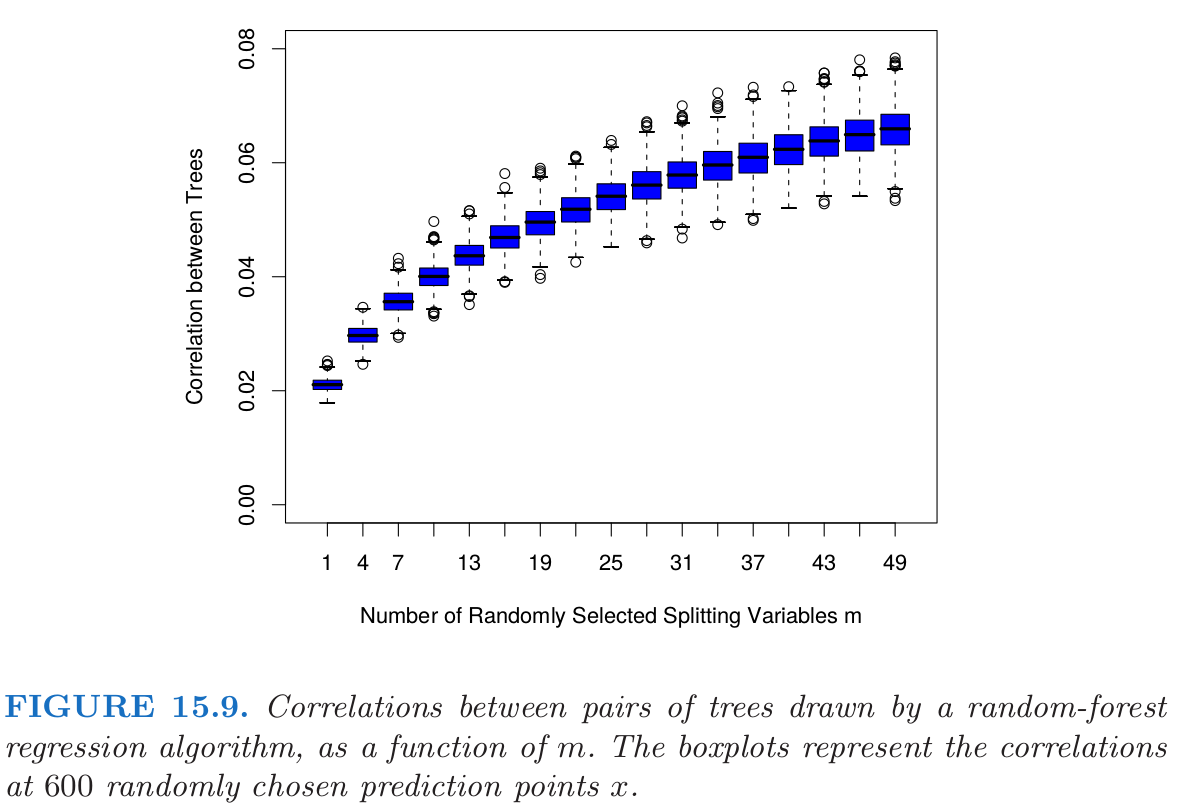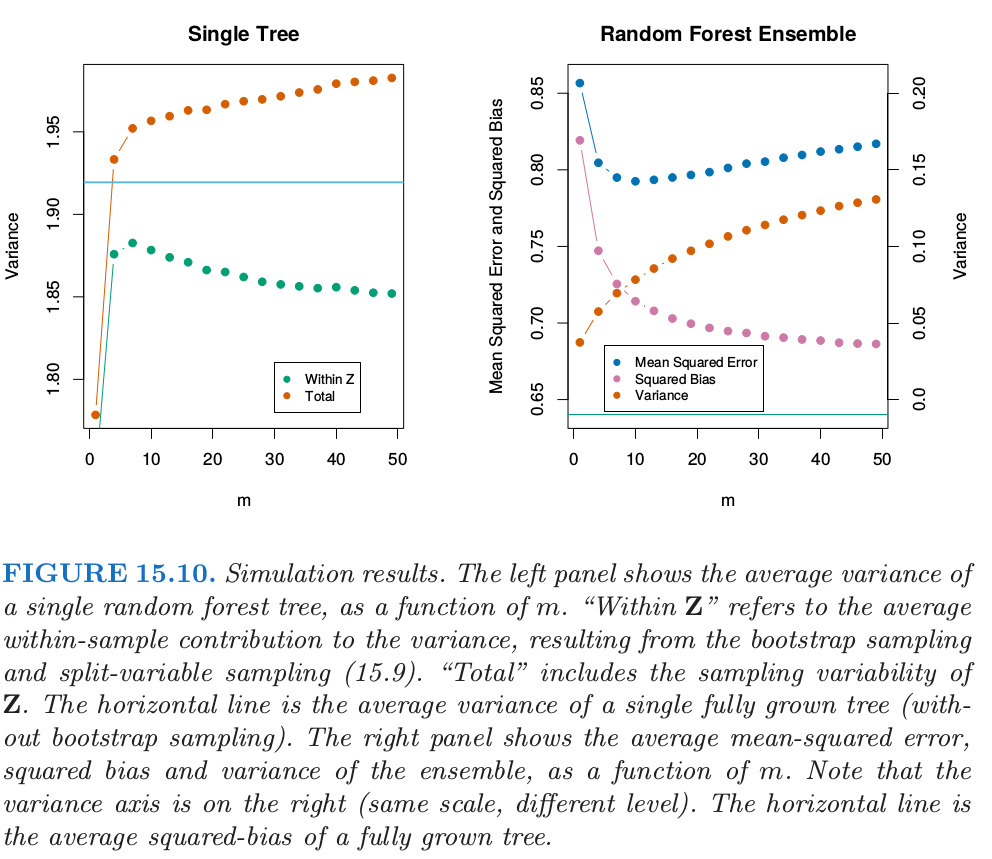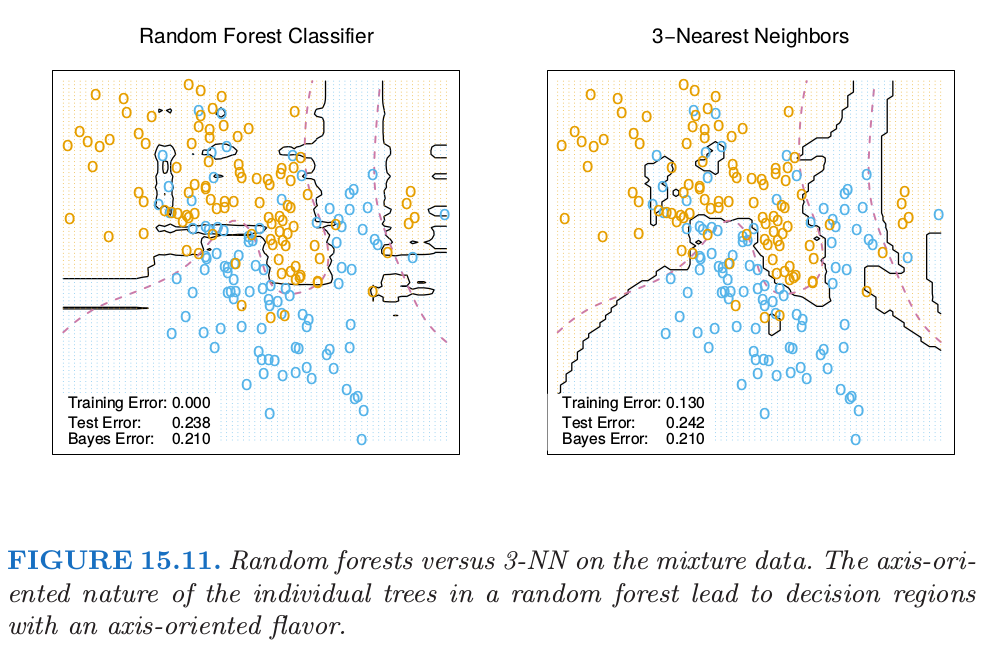15.4 随机森林的分析¶

方差和去相关性的影响¶

Recall

• $\rho(x)$ 是任意一对参与平均的树的的采样相关性: 其中 $\Theta_1(Z)$ 和 $\Theta_2(Z)$ 是随机抽取的一对随机森林树，这些树是根据 $Z^*$（$Z^*$ 是随机抽取 $Z$ 得到的自助样本）生成的．
• $\sigma^2(x)$ 是任意随机抽出的单棵树的采样方差

weiya注

weiya 注

• 既是在给定 $\mathbf Z$ 的条件下：因为自助采样和在每个分割点的特征采样
• 也是$\mathbf Z$自身的采样波动的结果

weiya 注： Ex. 15.5weiya 注： 条件方差公式

自适应最近邻¶